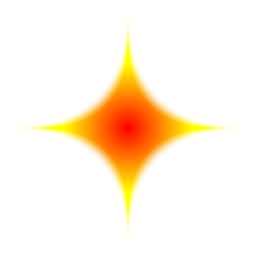Going round in squircles

October 29, 2012

If you were an N9 user, you might have noticed something about the shapes of many of the icons it used.

They weren't quite the usual squares, or even rectangles with rounded corners, but another shape called (I kid you not) a squircle, which if that wikipedia link is to be believed, is a kind of "superellipse".

Here's a picture of a squircle (in red) and a similar sized rounded rectangle (in green) so you can see the subtle differences: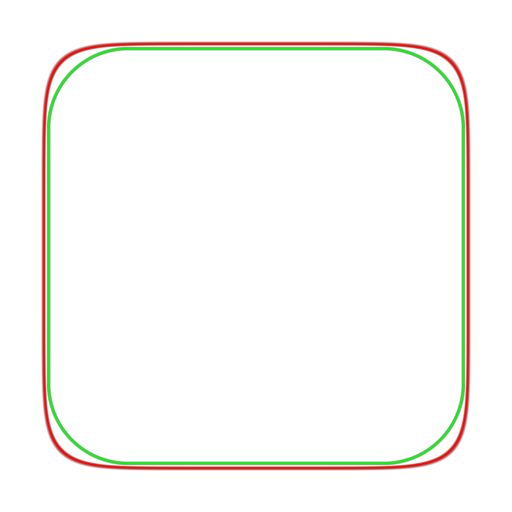The corners are less curved than the rounded rectangle.

I described earlier how to make a rounded rectangle shader in the Square shaped shaders article.

To make a squircle, again we start by using length, though it won't be quite a distance field we make. Wikipedia says we need to have a power of 4. So lets try this one:

f=vec4(1.-length(c.xy*c.xy*c.xy*c.xy));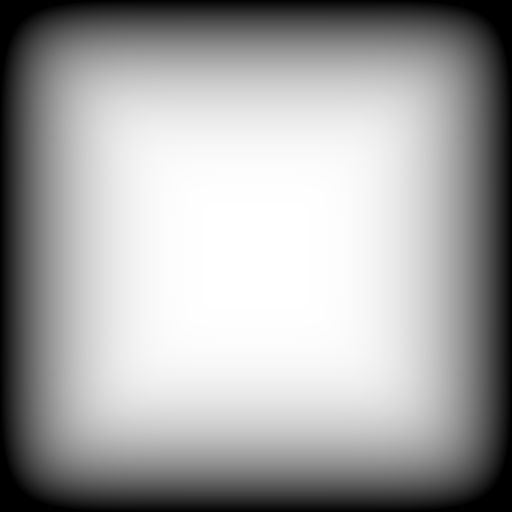It already looks quite squircley, doesn't it? That's before we've even applied a step function.

Here it is with the (smooth)step to make a solid shape with smooth edges:

float s=1.-length(c.xy*c.xy*c.xy*c.xy);
f=vec4(smoothstep(0.,0.1,s));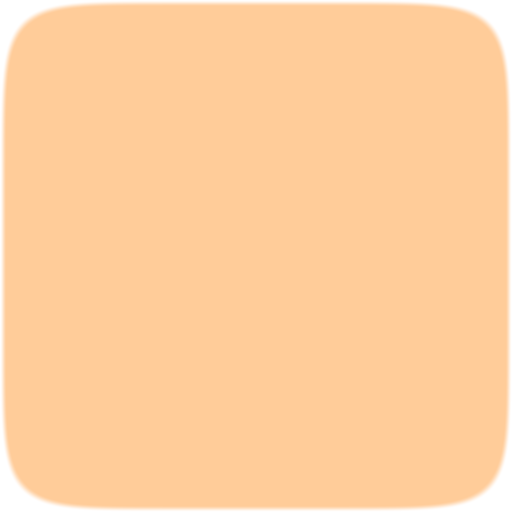I think it's quite nice - a bit more natural than plain rounded corners. It makes a nice mask or alpha channel for a PNG.

Here it is as the border for a beautifully calm and relaxing sky photo: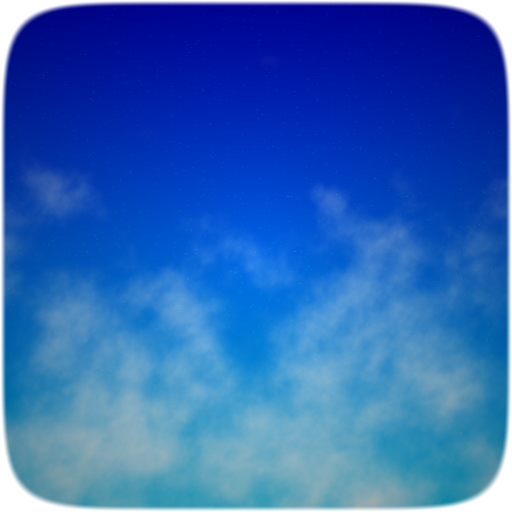Nah, that wasn't a photo after all, just another shader.

There is another way to write the squircle function which will lead us somewhere new. Instead of c.xy multiplied together 4 times, we can use the GLSL pow function, like this:

f=1.-length(pow(c.xy,vec2(4.)));

We already know that a power of 1 will give us a circle. Well, a large power will give us something very close to a square. But what about other numbers?

Here's a power of 3: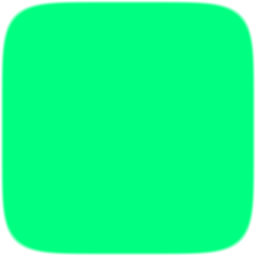Slightly curvier corners. Now a power of 2: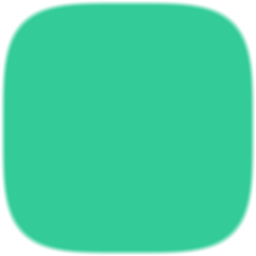Even curvier. Next 1.5 - yes, powers don't have to be integers: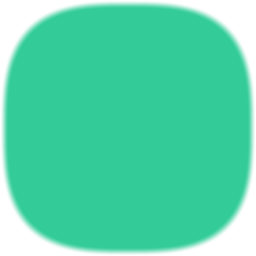Now 1.25: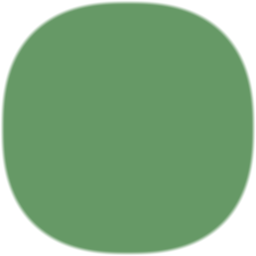We'll skip 1 - the circle. How about 0.85: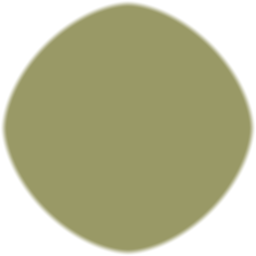0.5 is surprising: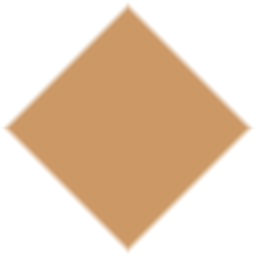A diamond!

And a last one to end with, 0.25: